Cross Multiplication Method

Chapter 3 Class 10 Pair of Linear Equations in Two Variables
Serial order wise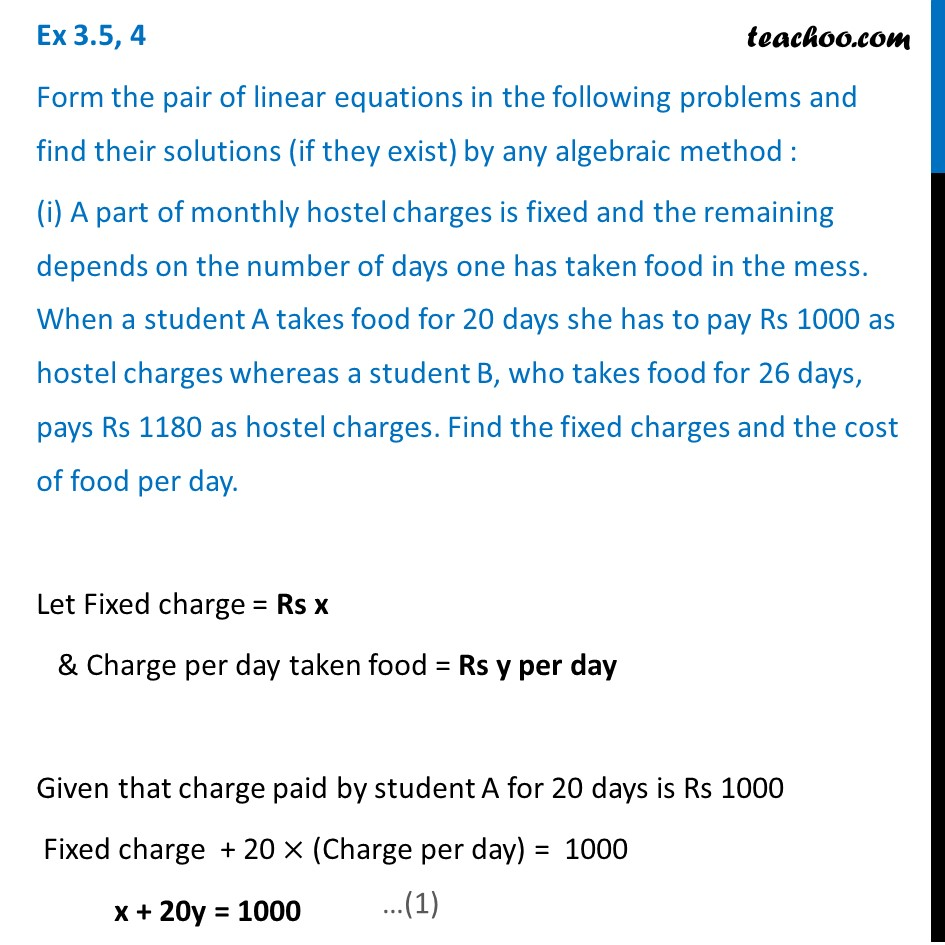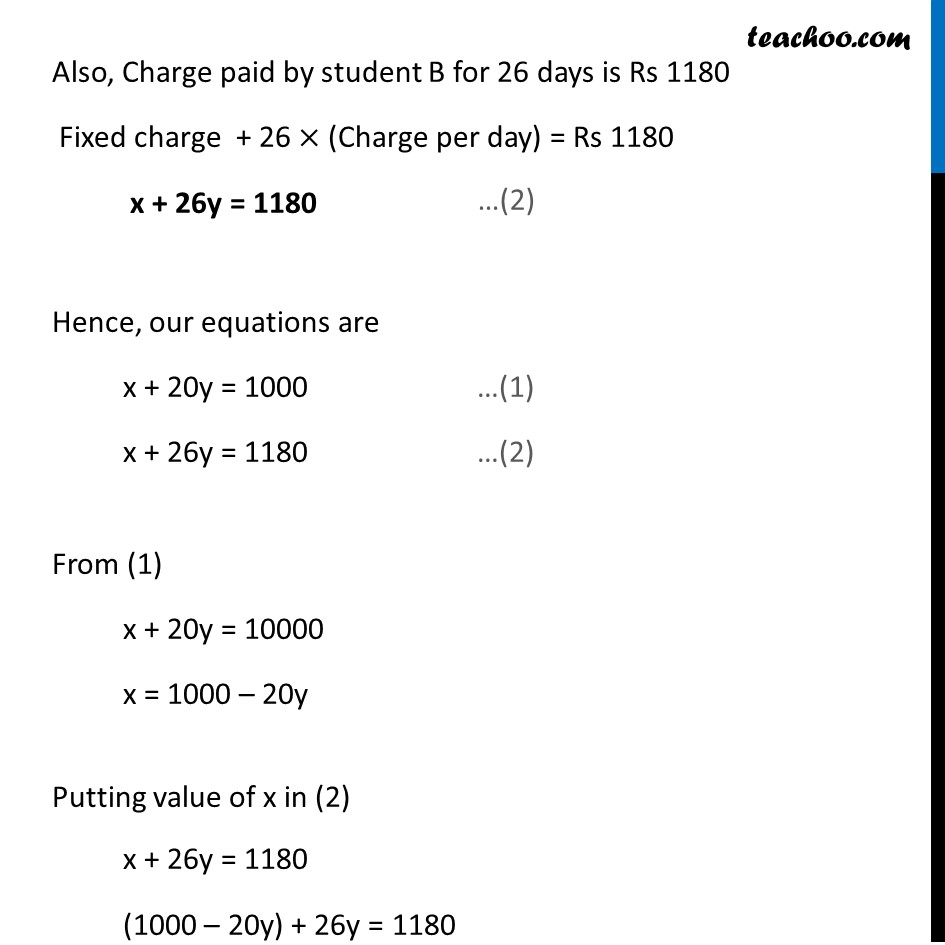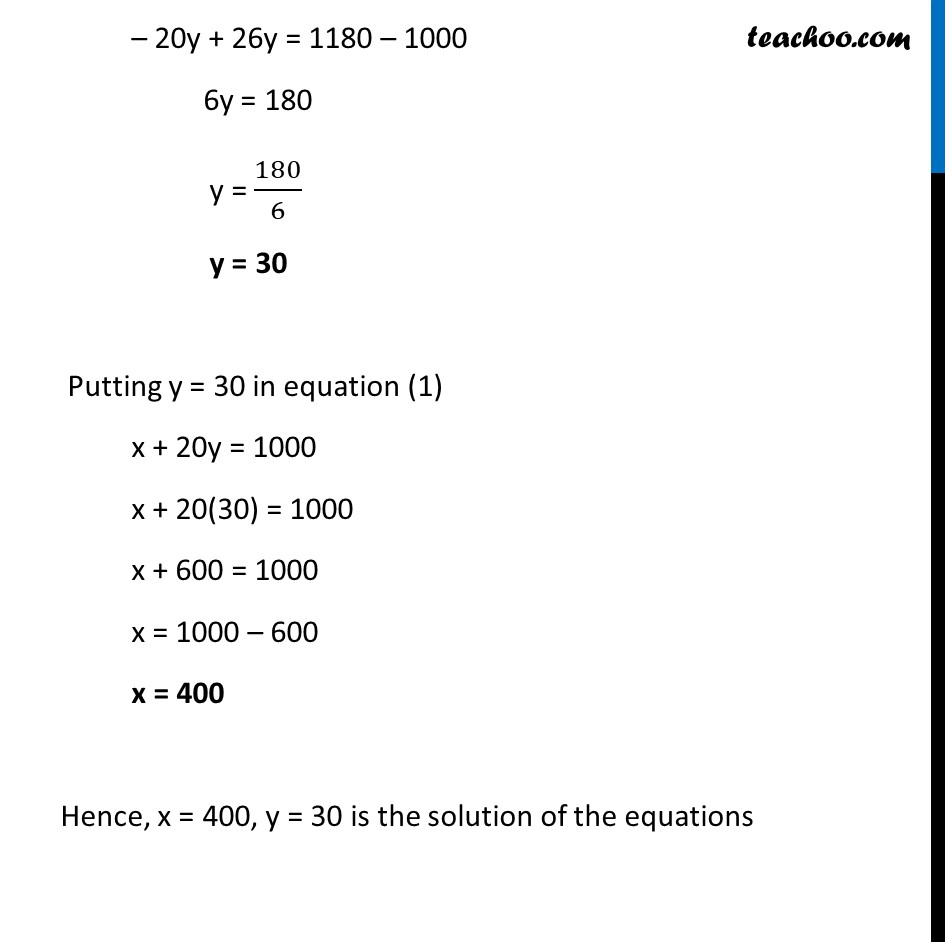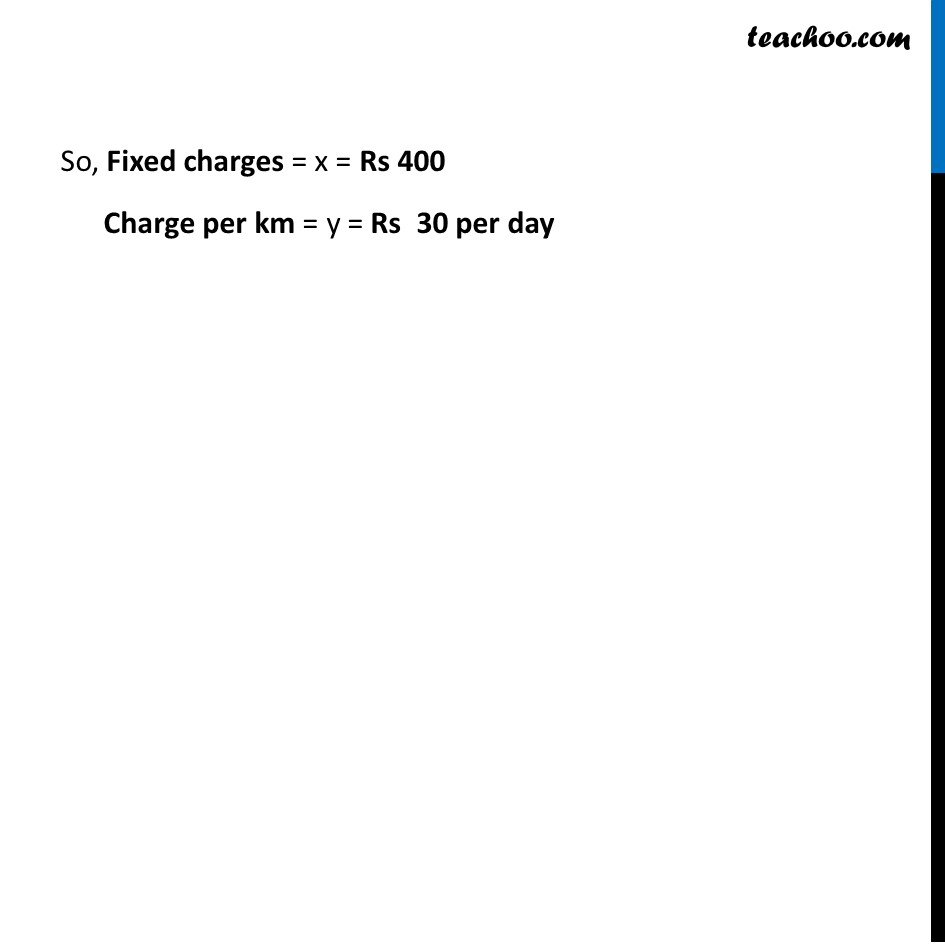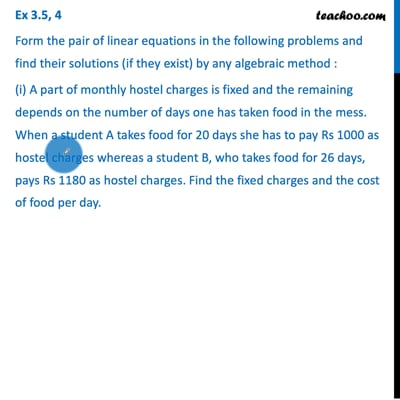This video is only available for Teachoo black users

Learn in your speed, with individual attention - Teachoo Maths 1-on-1 Class

### Transcript

Question 4 Form the pair of linear equations in the following problems and find their solutions (if they exist) by any algebraic method : (i) A part of monthly hostel charges is fixed and the remaining depends on the number of days one has taken food in the mess. When a student A takes food for 20 days she has to pay Rs 1000 as hostel charges whereas a student B, who takes food for 26 days, pays Rs 1180 as hostel charges. Find the fixed charges and the cost of food per day. Let Fixed charge = Rs x & Charge per day taken food = Rs y per day Given that charge paid by student A for 20 days is Rs 1000 Fixed charge + 20 × (Charge per day) = 1000 x + 20y = 1000 Also, Charge paid by student B for 26 days is Rs 1180 Fixed charge + 26 × (Charge per day) = Rs 1180 x + 26y = 1180 Hence, our equations are x + 20y = 1000 …(1) x + 26y = 1180 …(2) From (1) x + 20y = 10000 x = 1000 – 20y Putting value of x in (2) x + 26y = 1180 (1000 – 20y) + 26y = 1180 – 20y + 26y = 1180 – 1000 6y = 180 y = 180/6 y = 30 Putting y = 30 in equation (1) x + 20y = 1000 x + 20(30) = 1000 x + 600 = 1000 x = 1000 – 600 x = 400 Hence, x = 400, y = 30 is the solution of the equations So, Fixed charges = x = Rs 400 Charge per km = y = Rs 30 per day